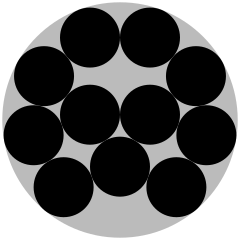homechevron_rightStudychevron_rightMathchevron_rightGeometry

# How many circles of radius r fit in a bigger circle of radius R

This calculator estimates how many circles of radius r can be placed inside another circle of radius R.This content is licensed under Creative Commons Attribution/Share-Alike License 3.0 (Unported). That means you may freely redistribute or modify this content under the same license conditions and must attribute the original author by placing a hyperlink from your site to this work https://planetcalc.com/7473/. Also, please do not modify any references to the original work (if any) contained in this content.

This calculator estimates the maximum number of smaller circles of radius r that fits into a larger circle of radius R. It could be the number of small pipes inside a large pipe or tube, the number of wires in a conduit, the number of cut circles from a circle-shaped plate, and so on.

One may think that there should be a formula for that, but, in fact, there is no formula. This is an optimization problem knows as Circle packing in a circle. It belongs to a class of optimization problems in mathematics, which are called packing problems and involve attempting to pack objects together into containers. Circle packing in a circle is a two-dimensional packing problem to pack unit circles into the smallest possible larger circle. See Circle packing in a circle.

For this problem, an optimal solution needs to be found and proved. Wikipedia article lists the first 20 solutions (in other words, it lists the smallest possible radius of the larger circle, which is enough to pack a specified number of unit circles (circles with a radius of one). Default calculator parameters, by the way, allow you to pack 11 circles, which will give you the layout presented below:http://en.wikipedia.org/wiki/File:Disk_pack11.svg

Happily, there is a project on the internet solely devoted to packing problems. It is the site called Packomania. Today, it summarises all found solutions. Site author Eckard Specht also participates in the search for solutions, and, in fact, most solutions were found by him, and there are solutions for up to 2600 circles in a large circle, with pictures of layouts. For each number of circles, the ratio of r/R is given, which can be used to find an answer.

The calculator below evaluates ratio r/R and then looks for the closest optimal solution among those 2600. If ratio r/R falls outside of research data, the calculator returns an error.#### How many circles of radius r fit in a bigger circle of radius R

Digits after the decimal point: 2
Number of small circles inside big

% used

URL copied to clipboardPLANETCALC, How many circles of radius r fit in a bigger circle of radius R